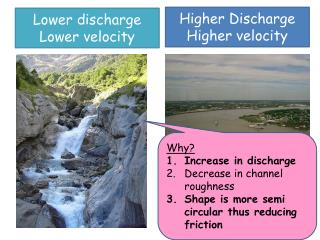DownloadDownload PresentationLower discharge Lower velocity

# Lower discharge Lower velocity

Télécharger la présentation## Lower discharge Lower velocity

- - - - - - - - - - - - - - - - - - - - - - - - - - - E N D - - - - - - - - - - - - - - - - - - - - - - - - - - -
##### Presentation Transcript

1. Higher Discharge Higher velocity Lower dischargeLower velocity Why? Increase in discharge Decrease in channel roughness Shape is more semi circular thus reducing friction

2. Calculating the Hydraulic Radius • To understand how to calculate the hydraulic radius of river channels • To summarise downstream changes

3. Hydraulic Radius • This measures the efficiency of a channel. • For an efficient channel you need less friction to occur. What shape river channel would you expect? • To calculate the hydraulic radius you need to be able to work out the; • Cross sectional area • Wetted perimeter

4. Cross sectional area = width x height E.g. 2m x 10m = 20m2 • Wetted Perimeter = the length of the cross section that is in touch with the water. You simply add these figures together. • E.g. 2m + 10m + 2m = 14metres

5. Hydraulic radius = cross sectional area / wetted perimeter E.g. 20 / 14 = 1.43 The higher the hydraulic radius the more efficient the channel is in carrying the water. The less friction there is!!

6. ACTIVITY Copy out the diagram on page 27 showing the changes in hydraulic radius downstream. Add cross sectional areas C and D. What do you notice about the hydraulic radius as you move downstream from A to D? Extension – can you create cross sectional area E?? Continue the pattern. C 5m x 7m D 8m x 10m

7. So what?! Why do we need to know how efficient a river is at carrying its water?

8. SUMMARY of downstream changes - What happens to the following list as you move downstream….. • Turbulence • Velocity • Friction • Discharge • Gradient • Size of load • Amount of load • Cross sectional area • Channel roughness • Hydraulic radius Downstream

9. SUMMARY of downstream changes - What happens to the following list as you move downstream….. • Turbulence • Velocity • Friction • Discharge • Gradient • Size of load • Amount of load • Cross sectional area • Channel roughness • Hydraulic radius Downstream